# 定义

## 零知识证明

### 定义

___比如：___有一个缺口环形的长廊,出口和入口距离非常近（在目距之内）,但走廊中间某处有一道只能用钥匙打开的门,A要向B证明自己拥有该门的钥匙。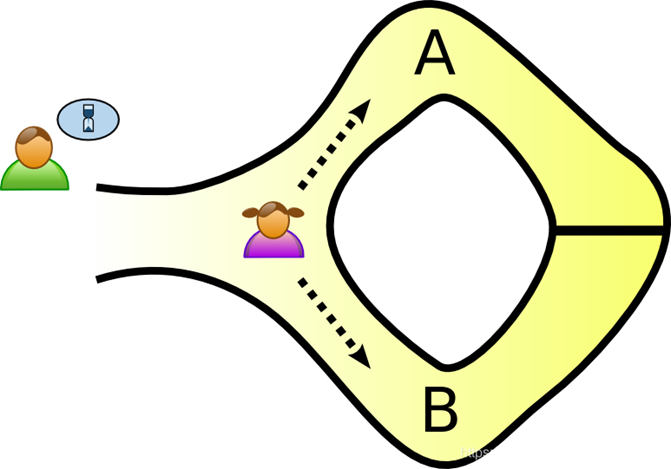### 性质

#### 完备性

P无法欺骗V。换言之,若P不知道一个定理的证明方法,则P使V相信他会证明定理的概率很低。

#### 正确性

V无法欺骗P。若P知道一个定理的证明方法,则P使V以绝对优势的概率相信他能证明。

V无法获取任何额外的知识。

# 历史

## 发展

• 1985~2010年,早年的零知识证明系统在效率以及可用性方面都有所欠缺,所以一直都停留在理论层面,直到最近10年才开始蓬勃发展,伴随着密码学在crypto成为显学,零知识证明走向台前,成为至关重要的一个方向。
特别是发展出一个通用的、非交互的、证明大小有限的零知识证明协议,是其中最关键的探索方向之一。
• 2010年,Groth实现了首个基于椭圆曲线双线性映射全能的,常数大小的非交互式零知识证明协议。后来这个协议经过不断优化,最终成为区块链著名的零知识证明协议SNARKs。
• 2013年,Pinocchio协议实现了分钟级别证明,毫秒级别验证,证明大小不到300字节,将零知识证明从理论带到了应用。后来Zcash使用的SNARKs正是基于Pinocchio的改进版。
• 2014 年,名为Zerocash的加密货币则使用了一种特殊的零知识证明工具zk-SNARKs （ Zero-Knowledge Succinct Non-interactive Arguments of Knowledge ) 实现了对交易金额、交易双方的完全隐藏,更注重于隐私,以及对交易透明的可控性。
• 2017 年, Zerocash 团队提出将 zk-SNARKs 与智能合约相互结合的方案,使交易能在众目睽睽下隐身,打造保护隐私的智能合约。

# 应用

## 解决区块链隐私问题

区块链将所有交易信息都放在了链上,即区块链的共识依靠区块链上的交易,而形成共识需要每个人都将交易下载下来并验证交易是正确的,因此区块链本身并不存在保密性。
如果使用零知识证明,可以在不泄露交易的情况下让别人验证交易是正确的。具体即是,每个矿工将自己的交易的零知识证明放到链上,而不是将交易直接放到链上。由于零知识证明的性质,如果证明正确则认为交易正确。

# 构建零知识证明

## 特殊情形

### 多项式承诺

#### 定义

• 承诺是一个公共值,它与提交者提供的原始信息绑定,提交者不会泄露消息。提交者需要打开此承诺并将消息发送到验证者,以验证承诺与消息之间的关系。
例如：PC可以被视为对某个多项式P的承诺,提交者可以证明多项式的值满足P(z)=a,而不会揭示多项式P。（个人理解,有一个多项式P,使用承诺可以在不泄露P的具体情况下,证明P(z)=a）
• 多项式承诺是一种最直观的零知识证明情形。

#### 多项式点值承诺

• 承诺生成阶段：证明者选择一个暂不公开的多项式,在某一点r处,计算出对应的承诺c并公开。c=f®,将(r,c)公开给验证者
• 承诺披露阶段：证明者公布多项式,验证者根据多项式计算r处值c’=f®,比较c’与c,一致则表示验证成功,否则失败。

如果公布多项式,则代表信息已经泄露,如何在不泄露具体信息的情况下,证明f®的值确实等于c呢？看起来确实无解。

#### 实用多项式点值承诺

• 承诺生成阶段：证明者选择一个暂不公开的多项式,在某一点r处,计算出对应的承诺c并公开。c=f®,将(r,c)公开给验证者。验证者需要验证的是,c=f®,但是验证者不知道f
• 挑战与证明生成阶段：验证者随机选择一个数z,发送给证明者P,P计算在z的值s=f（z）,同时计算出t(x)=f(x)-s/(x-z),计算t(x)在z处的值w=t(z),返回给验证者V(s,w)。
• 验证阶段：
1.验证者需要验证s=f(z)
2.验证者需要验证f(z)-s=0 -> 即f(x)-s=0,有根z
3.存在t(x)使得f(x)-s=t(x)(x-z),这个等式是恒等式,所以任意点成立。到此处,可以证明该等式确实和原式相关？
总结：对于多项式承诺的验证,不需要公布多项式,只需要对于任意的验证者给出的z,证明者能够给出一个数值对（s,w),同时f(z)-s=w(z-z),这便是一次零知识证明的交互
前三步证明了一个事情,即f(x)-z=t(x)(x-z),可以得出f与t有倍数关系,这便是s=zw,只要对于任意给出的z,返回的(s,w)都可以得出s=zw,便可以认为该承诺成立。
4.因为该等式在任意点成立,所以在r处成立,所以可以验证f®-s=t®(r-z)=c-s=w(r-z)
原理为：验证者验证p=t*h,如果多项式相等,意味着t(x)是p(x)的因式。只要P猜中r的概率不超过e,如果这是一个非常小的值,则可以认为这是一个计算意义上的完备。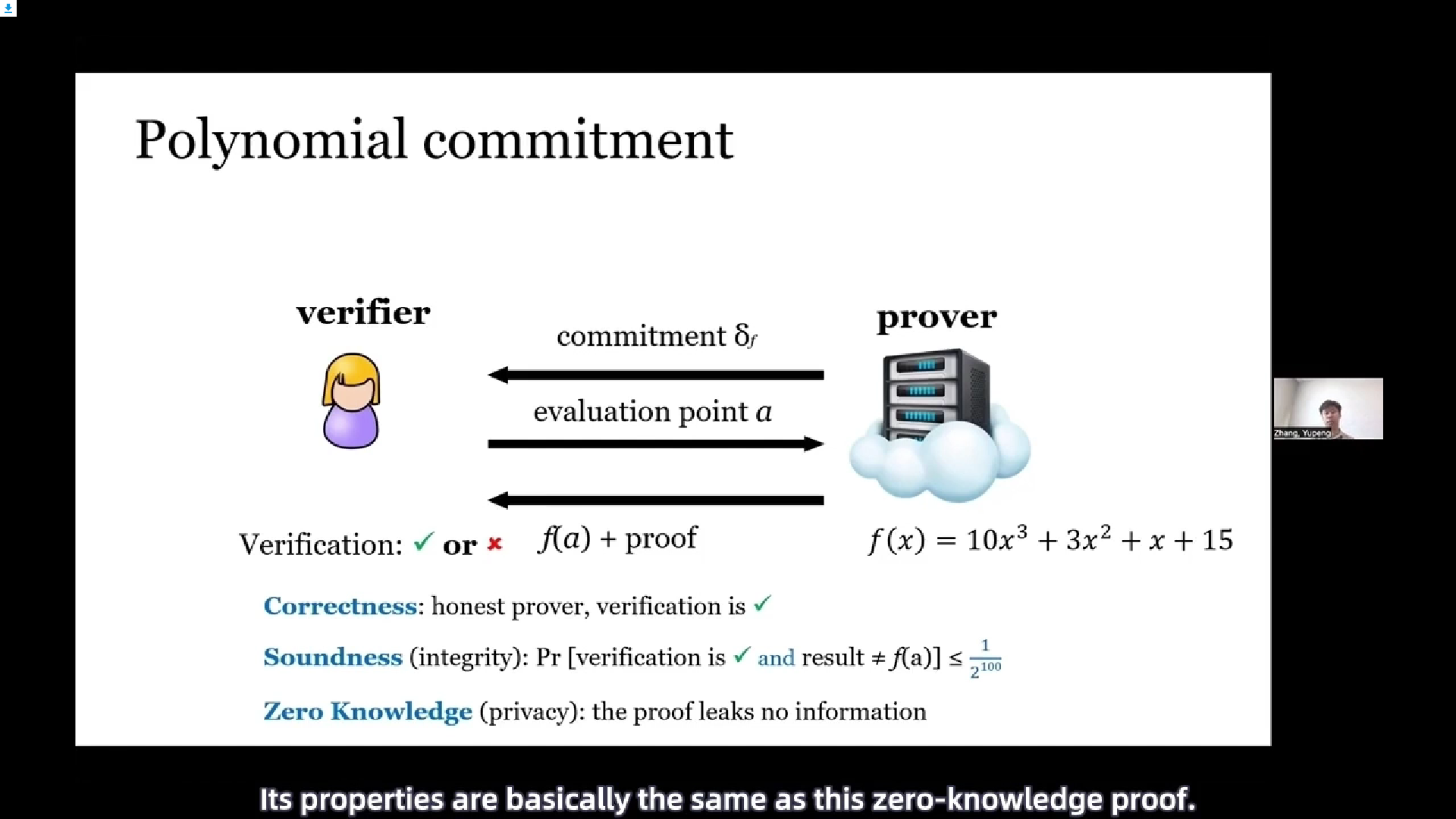##### 存在的问题

证明者可能并不知道他所宣称的p(x),他可以先算一下t=t(r ),然后选择一个随机值h,由此计算出p=t*h。因为等式是成立的,所以也能通过验证者的校验。 因为证明者知道随机点x=r,他可以构造出一个任意的多项式,这个任意多项式与t®*h®在r处有共同点。

##### 解决方法
• 证明者不可以直接得到验证者查询的随机数
• 必须约束这个多项式阶数在一定范围内

### 多项式承诺的零知识证明

• PLONK
• 交互式证明
• DARK
• Stark（low degree cost）

### 前置知识

• 质数和模运算
• 拉格朗日插值
• 生成元
通过对生成元的操作,可以遍历整个群,即将有限域里面的所有数写成指数形式。
• 双线性映射（Bilinear map）
在数论中,一个双线性映射是由两个向量空间上的元素,生成第三个向量空间上一个元素之函数,并且该函数对每个参数都是线性的。例如矩阵乘法

B:VxW->X // 这是一个映射关系

### KZG

KZG多项式承诺是Kate, Zaverucha 和 Goldberg 等人在 2010 年发表的 Polynomial Commitments 论文提出的对多项式进行承诺的一种方案. 目前而言, 区块链上许多项目准备使用 KZG 多项式承诺解决一些特定问题, 例如 EIP-4844 以及零知识证明相关项目.

Univariate polynomial commitment 单变量多项式承诺

#### 可信设置

在使用KZG多项式承诺之前, 需要先进行可信设置(Trust setup). 在这个步骤中, 首先生成无人知晓的s,s∈Fr,并计算sⁱ⋅G₁和sⁱ⋅G₂,i=1,…,随后销毁s.

• s为无人知晓的私钥,在计算出 sⁱ⋅G₁和sⁱ⋅G₂之后立即销毁
• sⁱ⋅G₁ 和 sⁱ⋅G₂ 公开, 每个人都知道

#### 卡特承诺

多项式可以表达为以下结构f(x) = a₀⋅x⁰ + a₁⋅x¹ + a₂⋅x² … + aₙ⋅xⁿ
因此我们假设该多项式的承诺为C = f(s)⋅G₁ = a₀⋅s⁰⋅G₁ + a₁⋅s¹⋅G₁ + a₂⋅s²⋅G₁ … + aₙ⋅sⁿ⋅G₁

• 公开的参数：p,g
• 密钥：s
• 公钥：$g,g^s,g^(s^2),g^(s^3),...,g^(s^d)$
1.prover -> commitment g^f(s)
2.verifier -> evaluation point a
3.prover -> v=f(a) proof=$g^q(s)$所以证明者还要将零知识的证明方法发给验证者,用以验证。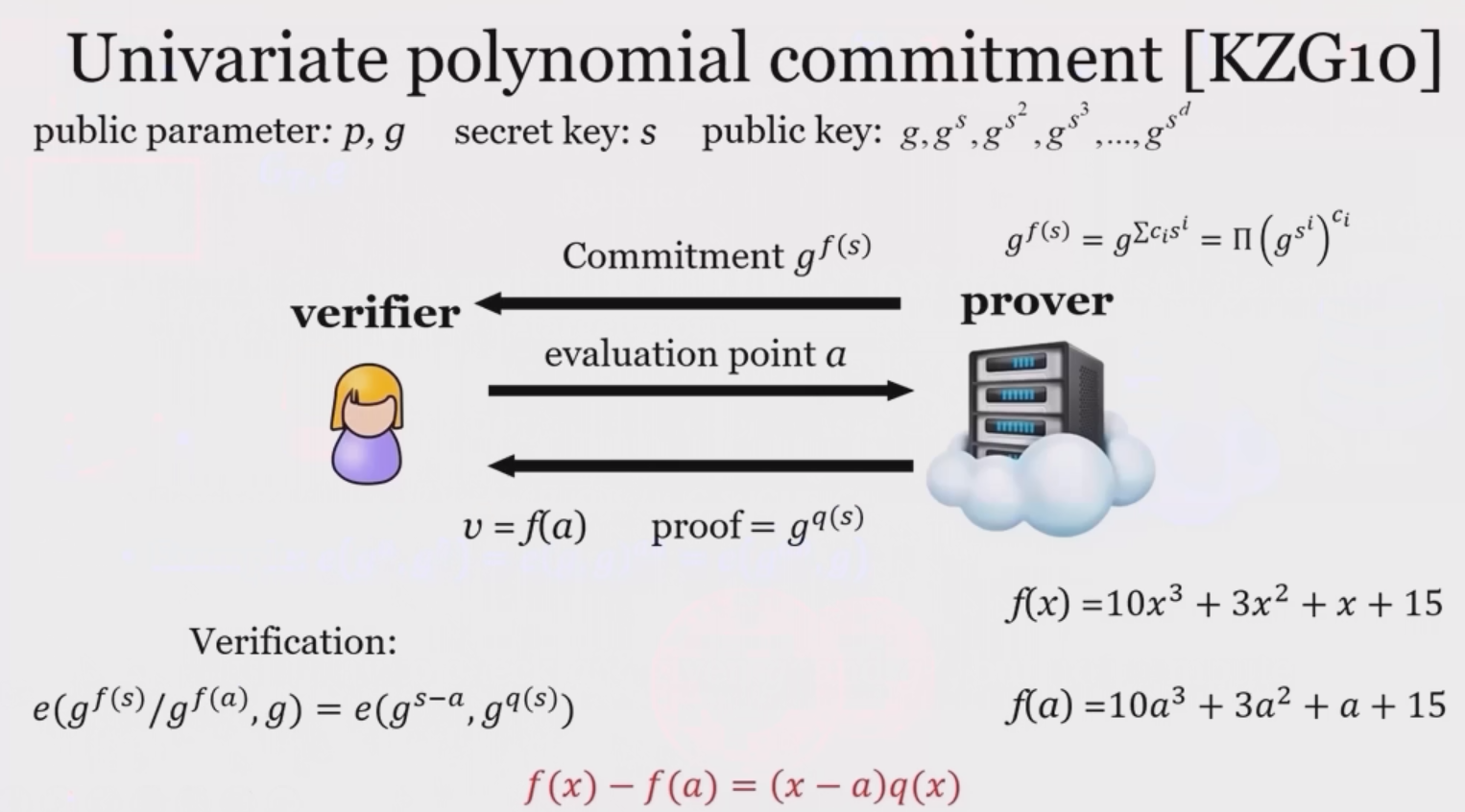#### 复杂度问题

• 承诺：O(1)
• 证明时间：O(d)
• 证明大小：O(1) ~100 bytes
• 验证：O(1) 0.3ms

## 一般情形

### Generic ZKP

• 将任意的zkp都放到电路上

### PLONK

• Plonk circuit to constraint system
Gate constraint

#### PLONK工作原理

PLONK的一个关键要素是,将问题的形式从“给我一个值x,我给你一个特定的程序p,这样当x作为输入进行计算的时候,我给你一些具体的结果Y”变成了“给我一组值”,这个就和zk-snark中的QAP一样。（从这里看是从给一个值扩展到了给一组值）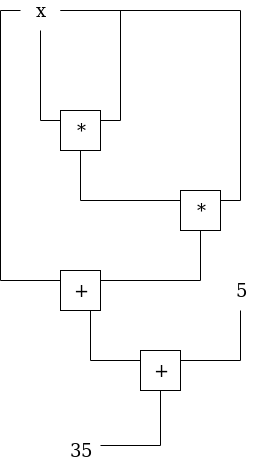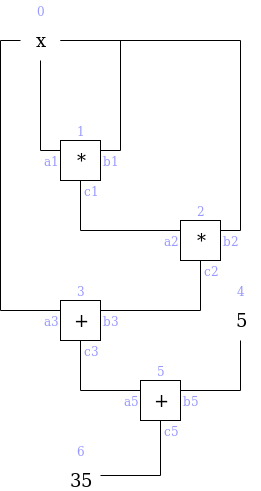\begin{aligned} \left(Q_{L_{i}}\right) a_{i}+\left(Q_{R_{i}}\right) b_{i}+\left(Q_{O_{i}}\right) c_{i}+\left(Q_{M_{i}}\right) a_{i} b_{i}+Q_{C_{i}}=0 \end{aligned}

#### 线性系统到多项式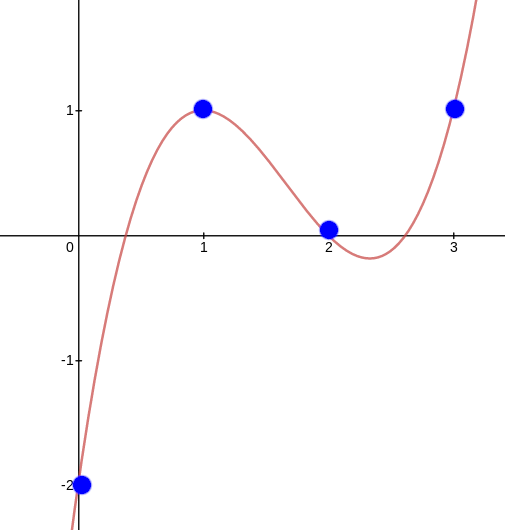\begin{aligned} \begin{array}{l}{2 x_{1}-x_{2}+3 x_{3}=8} \\ {x_{1}+4 x_{2}-5 x_{3}=5} \\ {8 x_{1}-x_{2}-x_{3}=-2}\end{array} \end{aligned}

\begin{aligned} L(x) \cdot x_{1}+M(x) \cdot x_{2}+R(x) \cdot x_{3}-O(x)=Z(x) H(x) \end{aligned}

\begin{aligned} Q_{L}(x) a(x)+Q_{R}(x) b(x)+Q_{O}(x) c(x)+Q_{M}(x) a(x) b(x)+Q_{C}(x)=0 \end{aligned}

#### 复制约束

a(5)=c(7),c(10)=c(12)等等。这需要一些巧妙的技巧。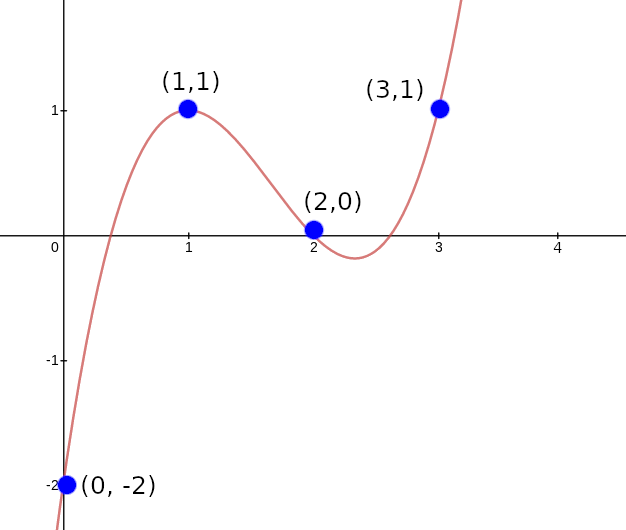1 2 3 4 5 6
$X(x)$ 0 1 2 3 4
$Y(x)$ -2 1 0 1
$v_1+X(x)+v_2Y(x)$ -1 6 5 8 1
$p(x)$ 1 -1 -6 -30 -240

我们发现p(4)=-240.现在考虑用X(x)=x来进行替代,我们设$X(x)=\frac{2}{3}x^3-4x^2+\frac{19}{3}x$（这样多项式在(0,1,2,3)的值是(0,3,2,1)）如果你运行同样的程序,你会发现你也能得到p(4)=-240。这并不是一个巧合（实际上,如果你从特别大的域里面随机选择$v_1$$v_2$,它也不会发生这样的巧合）

\begin{aligned} p_{a}(n) \cdot p_{b}(n) \cdot p_{c}(n)=p_{a}^{\prime}(n) \cdot p_{b}^{\prime}(n) \cdot p_{c}^{\prime}(n) \end{aligned}

#### 整合所有后

$p(x + 1) = p(x) \cdot (v_1 + X(x) + v_2 \cdot Y(x))$转变成 $p(\omega \cdot x) = p(x) \cdot (v_1 + X(x) + v_2 \cdot Y(x))$而不是使用$0..n-1$,$n...2n-1$,$2n..3n-1$作为坐标对,我们使用g是领域中一些随机的高阶元素时的\omega ^,$g \cdot \omega ^2$$g^2\omega ^2$
$emsp;$emsp;现在让我们写下我们需要检查的所有等式。首先时主要的门电路满足度检查：

\begin{aligned} Q_{L}(x) a(x)+Q_{R}(x) b(x)+Q_{O}(x) c(x)+Q_{M}(x) a(x) b(x)+Q_{C}(x)=0 \end{aligned}

\begin{aligned} \begin{array}{l}{P_{a}(\omega x)-P_{a}(x)\left(v_{1}+x+v_{2} a(x)\right) =Z(x) H_{1}(x)} \\ {P_{a^{\prime}}(\omega x)-P_{a^{\prime}}(x)\left(v_{1}+\sigma_{a}(x)+v_{2} a(x)\right)=Z(x) H_{2}(x)} \\ {P_{b}(\omega x)-P_{b}(x)\left(v_{1}+g x+v_{2} b(x)\right)=Z(x) H_{3}(x)} \\ {P_{b^{\prime}}(\omega x)-P_{b^{\prime}}(x)\left(v_{1}+\sigma_{b}(x)+v_{2} b(x)\right)=Z(x) H_{4}(x)} \\ {P_{c}(\omega x)-P_{c}(x)\left(v_{1}+g^{2} x+v_{2} c(x)\right)=Z(x) H_{5}(x)} \\ {P_{c^{\prime}}(\omega x)-P_{c^{\prime}}(x)\left(v_{1}+\sigma_{c}(x)+v_{2} c(x)\right)=Z(x) H_{6}(x)}\end{array} \end{aligned}

\begin{aligned} \begin{array}{l}{P_{a}(1)=P_{b}(1)=P_{c}(1)=P_{a^{\prime}}(1)=P_{b^{\prime}}(1)=P_{c^{\prime}}(1)=1} \\ {P_{a}\left(\omega^{n}\right) P_{b}\left(\omega^{n}\right) P_{c}\left(\omega^{n}\right)=P_{a^{\prime}}\left(\omega^{n}\right) P_{b^{\prime}}\left(\omega^{n}\right) P_{c^{\prime}}\left(\omega^{n}\right)}\end{array} \end{aligned}

• 线分配为$a(x),b(x),c(x)$
• 坐标对累加器$P_a(x), P_b(x), P_c(x), P_{a'}(x), P_{b'}(x), P_{c'}(x)$
• $H(x)$$H_1(x)...H_6(x)$
证明者和验证者首先需要计算的程序指定的多项式是：
• $Q_L(x), Q_R(x), Q_O(x), Q_M(x), Q_C(x)$,这些东西代表了电路中的所有门（$Q_C(x)$加密公共输入,因此它可能需要在运行时加密或修改）
• “排列多项式”$\sigma_a(x), \sigma_b(x)$$\sigma_c(x)$,加密了a,b,c线中的复制约束

#### 回顾

\begin{aligned} \left(Q_{L_{i}}\right) a_{i}+\left(Q_{R_{i}}\right) b_{i}+\left(Q_{O_{i}}\right) c_{i}+\left(Q_{M_{i}}\right) a_{i} b_{i}+Q_{C_{i}}=0 \end{aligned}

\begin{aligned} Q_{L}(x) a(x)+Q_{R}(x) b(x)+Q_{O}(x) c(x)+Q_{M}(x) a(x) b(x)+Q_{C}(x)=0 \end{aligned}

\begin{aligned} Q_{L}(x) a(x)+Q_{R}(x) b(x)+Q_{O}(x) c(x)+Q_{M}(x) a(x) b(x)+Q_{C}(x)=0 \end{aligned}

# 热门协议

## zk-SNARKs

1.可扩展性：如果一个区块链需要长时间来验证,一个人可以验证它并生成证明,而其他人可以快速验证证明
2.隐私：您可以证明您有权转移某些财产,而无需透漏您收到的资产的链接。这确保了安全性,而不会向公众过度泄漏有关谁与谁在进行交易的信息。

### 多项式

• $x+5$
• $x^4$
• $x^3 + 3x^2 + 3x + 1$
• $628x^{271} + 318x^{270} + 530x^{269} + ... + 69x + 381$

• $A(0) + B(0) = C(0)$
• $A(1) + B(1) = C(1)$
• $A(2) + B(2) = C(2)$

• $12+1=13$
• $10+8=18$
• $15+8=23$
• $15+13=28$

• $A(x) = -2x^3 + \frac{19}{2}x^2 - \frac{19}{2}x + 12$
• $B(x) = 2x^3 - \frac{19}{2}x^2 + \frac{29}{2}x + 1$
• $C(x) = 5x + 13$

### 多项式承诺

• 加法 给定一个com§,com(Q)和com®,检查P+Q=R是否成立
• 乘法 给定一个com§,com(Q)和com®,检查P*Q=R是否成立
• 计算点值 给定一个com§,w,z和一个补充证明(或者“证人”)Q,验证P(w)=z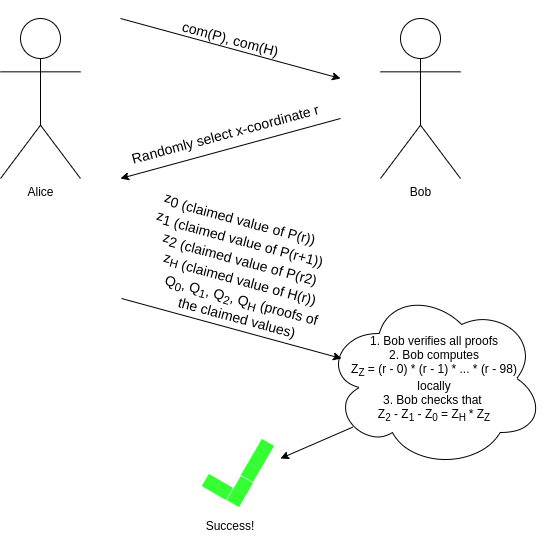### 快速回顾

• zk-SNARKs很难,因为验证者需要以某种方式检查计算中的数百万步,而不需要做一些工作来直接检查每个单独的步骤（因为这会花费太长时间）.
• 我们通过将计算编码为多项式来解决这个问题
• 单个多项式可以包含无限大量的信息,并且单个多项式（例如 P(x+2) - P(x+1) - P(x) = Z(x) * H(x)）可以"代替"数字之间无限数量的方程.
• 如果可以用多项式验证方程,则可以隐式验证所有数字方程（使用任意x坐标来代替x）
• 我们使用多项式的一种特殊类型的"哈希",称为多项式承诺,这可以使我们在很短的时间内实际验证多项式之间的方程,即使底层多项式非常大。

### FRI工作方式

• P(x)实际上等于$Q(x^2) + x * R(x^2)$
• S(x)实际上等于$Q(x) + r * R(x)$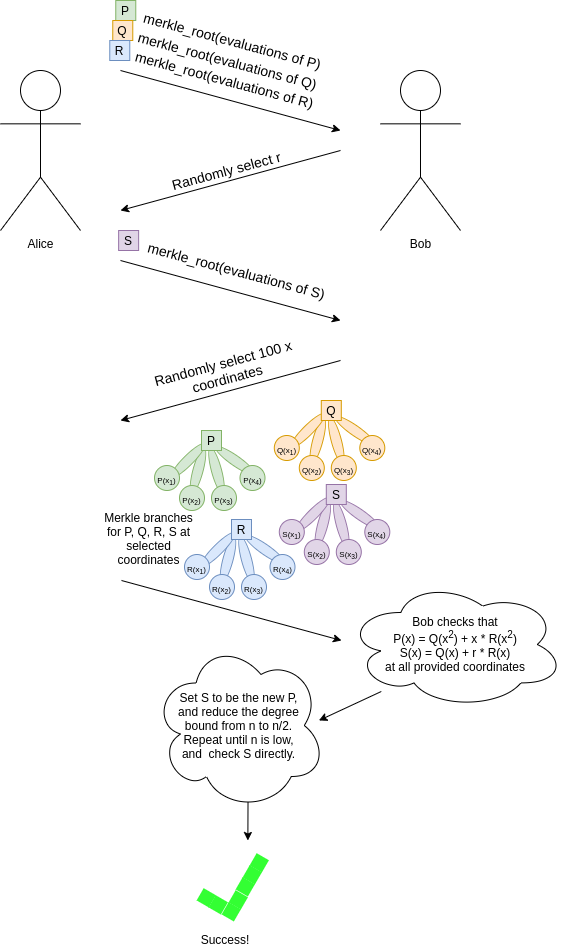1.默克尔树根点P
2.默克尔树根点Q,R,$S_1$
3.是随机选择的分支P,Q,R,$S_1$要检查$S_1$正确地减少自P
4.默克尔根和随机选择的分支,如步骤(2)和(3)中用于连续降低度数的还原$S_2$$S_1,S_3$$S_2$,所有的降低到低的读书$S_k$（总共可以重复$log_2 n$次）
5.全部的默克尔树的点$S_k$

### 有限域

$x + y \Rightarrow (x + y)$

$x * y \Rightarrow (x * y)$

$x^y \Rightarrow (x^y)$

$x - y \Rightarrow (x - y)$

$x / y \Rightarrow (x * y ^{p-2})$

5 + 3 = 1($$作为$$8%7=1)

1 - 3 = 5($$作为$$-2%7=5)

$2 \cdot 5 = 3$

3 / 5 = 2$$（如$$(3 \cdot 5^5)%7=9375%7=2）

### 例子

• P(0)=0
• P(x+1) = P(x)*2+R(x) 范围为{0…63}
• $R(x) \in \{0,1\}$ 范围为{0…63}

• $P(x+1) - P(x) * 2 - R(x) = Z(x) * H_1(x)$

• R(x) * (1 - R(x)) = Z(x) * H_2(x)（注意 当且仅当$y \in \{0, 1\}$时,$y * (1-y) = 0$）

### 再回顾

• 三种最突出的承诺类型是FRI、Kate和bulletproof
• Kate在概念上是最简单的,但它取决于椭圆曲线配对的真正复杂度“黑匣子”
• FRI很酷,因为它只依赖于哈希；它的工作原理是连续将多项式简化为低次多项式,并使用默克尔分支进行随机样本检查以证明每一步的等价性。
• 为了防止单个数的大小爆炸,我们不是在整数域上进行算术和多项式,而是在有限域上做所有事情（通常是整数模一些素数p）
• 多项式承诺自然而然地有助于隐私保护,因为证明比多项式小得多,因此多项式承诺无论如何都不能透漏多项式中多一点点信息。但是我们可以为承诺的多项式添加一些随机性,以将泄露的信息从“一点点”减少到“零”

### 哪些问题仍在研究中？

• 优化FRI：已经有相当多的优化设计及精心选择的域计算、“DEEP-FRI”以及许多其他技巧,以使FRI更高校。Starkware和其他人正在研究这个问题
• 将计算编码为多项式的更好方法：找出将涉及哈希函数、内存访问和其他特征的复杂计算编码为多项式方程的最有效方法仍然使一个挑战。在这方面已经取得了很大进展（例如,PLLOKUP）,但我们仍然需要更多,特别是如果我们想将通用虚拟机执行编码为多项式
• 可增量验证的计算：能够在计算继续进行的同时有效地“扩展”证明,那就太好了。这在“单证明者”案例中很有价值,但在“多证明者”案例中也很有价值,特别是区块链,其中不同的参与者创建每个区块。